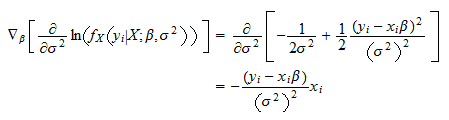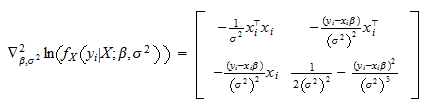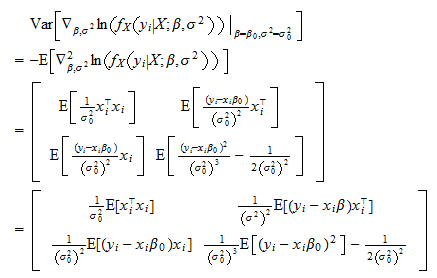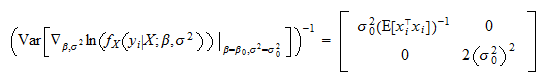StatLect

# Linear regression - Maximum Likelihood Estimation

This lecture shows how to perform maximum likelihood estimation of the parameters of a Normal Linear Regression Model, that is, of a linear regression model whose error terms are normally distributed conditional on the regressors.

In order to fully understand the material presented in this lecture, it might be useful to revise the lectures on Maximum likelihood estimation and on the Normal Linear Regression Model.## Assumptions

The objective is to estimate the parameters of the linear regression modelwhereis the dependent variable,is avector of regressors,is thevector of regression coefficients to be estimated andis an unobservable error term.

We assume that our sample is made up ofIID observations.

The regression equations can be written in matrix form aswhere thevector of observations of the dependent variable is denoted by, thematrix of regressors is denoted by, and thevector of error terms is denoted by.

We also assume that the vector of errorshas a multivariate normal distribution conditional on, with mean equal toand covariance matrix equal towhereis theidentity matrix andNote that alsois a parameter to be estimated.

Furthermore, it is assumed that the matrix of regressorshas full-rank.

The assumption that the covariance matrix ofis diagonal implies that the entries ofare mutually independent (i.e.,is independent offor.). Moreover, they all have a normal distribution with meanand variance.

By the properties of linear transformations of normal random variables, we have that also the dependent variableis conditionally normal, with meanand variance. Therefore, the conditional probability density function of the dependent variable is## The likelihood function

The likelihood function isProof

Since the observations from the sample are independent, the likelihood of the sample is equal to the product of the likelihoods of the single observations:## The log-likelihood function

The log-likelihood function isProof

It is obtained by taking the natural logarithm of the likelihood function:## The maximum likelihood estimators

The maximum likelihood estimators of the regression coefficients and of the variance of the error terms areProof

The estimators solve the following maximization problemThe first-order conditions for a maximum arewhereindicates the gradient calculated with respect to, that is, the vector of the partial derivatives of the log-likelihood with respect to the entries of. The gradient iswhich is equal to zero only ifTherefore, the first of the two equations is satisfied ifwhere we have used the assumption thathas full rank and, as a consequence,is invertible. The partial derivative of the log-likelihood with respect to the variance iswhich, if we assume, is equal to zero only ifThus, the system of first order conditions is solved byNote thatdoes not depend on, so that this is an explicit solution.

Thus, the maximum likelihood estimators are:

1. for the regression coefficients, the usual OLS estimator;

2. for the variance of the error terms, the unadjusted sample variance of the residuals.

## Asymptotic variance

The vector of parametersis asymptotically normal with asymptotic mean equal toand asymptotic covariance matrix equal toProof

The firstentries of the score vectorareThe-th entry of the score vector isThe Hessian, that is, the matrix of second derivatives, can be written as a block matrixLet us compute the blocks:andFinally,Therefore, the Hessian isBy the information equality, we have thatButand, by the Law of Iterated Expectations,Thus,As a consequence, the asymptotic covariance matrix isThis means that the probability distribution of the vector of parameter estimatescan be approximated by a multivariate normal distribution with meanand covariance matrixThe book

Most of the learning materials found on this website are now available in a traditional textbook format.

Glossary entries
Share